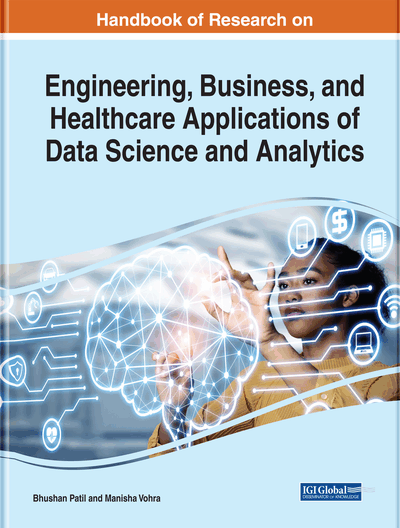# Data Analysis in Context-Based Statistical Modeling in Predictive Analytics

DOI: 10.4018/978-1-7998-3053-5.ch006
OnDemand:
(Individual Chapters)
Available
\$37.50
No Current Special Offers

## Abstract

Data analysis is a process of studying, removing non-required data in the view level, and converting to needed patterns for sub decisions to make an aggregated decision. Statistical modeling is the process of applying statistical techniques in data analysis for taking proactive decisions depend requirements. The statistical modeling identifies relationship between variables, and it encompasses inferential statistics for model validation. The focus of the chapter is to analyze statistical modeling techniques in different contexts to understand the mathematical representation of data. The correlation and regression are used for analyzing association between key factors of companies' activities. Especially in business, correlation describes positive and negative correlation variables for analyzing the factors of business for supporting the decision-making process. The key factors are related with independent variables and dependent variables, which create cause and effect models to predict the future outcomes.
Chapter Preview
Top

## Key Terms in this Chapter

Bivariate Analysis: It is one of the statistical analyses where two variables are involved to figure out the depth of the relationships between variables.

Data Analytics: The science of extracting meaningful information continuously with the assistance of specialized system for finding patterns to get feasible solutions.

Regression Analysis: It is a statistical process for denoting the average relationship between two or more factors with the involvement of dependent and independent variables.

Statistical Modeling: It represents a mathematical model which encompasses statistical assumptions to interpret the available data for approximating reality.

Multivariate Analysis: It is a subdivision of statistics for analyzing three or more piece of information for every item to find the interrelationship between variables to predict the future outcomes.

Predictive Analytics: The finding of new patterns is to analyze future performance of a system based on context and historical information and it is used for decision making process.

Univariate Analysis: It is a simplest form of statistical analysis which involves with single variable for finding solution in predictive analytics.

Correlation: The degree of relationship between two or more variables and it does not reflect cause and effect relationship between the factors.

## Complete Chapter List

Search this Book:
Reset